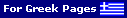Antenna Circular Polarization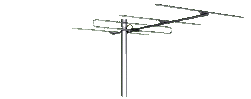Space communication has forced the use of Circular polarization. The fundamental advantage of circular polarization is that all reflections change the direction of polarization, precluding the usual addition or subtraction of main and reflected signals. Therefore there is far less fading and flutter when circular polarization is used at each end of the link.

The use of circular polarization at one end only gives a loss of about -3dB. In order to achieve the full advantages of circular polarization it is necessary for all stations to use it. The table below shows the relationship between Horizontal, Vertical, RHCP (Right Hand Circular Polarization) & LHCP (Left Hand Circular Polarization) and Loss in dB. RHCP is also known as CW (ClockWise) Polarization and LHCP as CCW (Counter ClockWise) Polarization.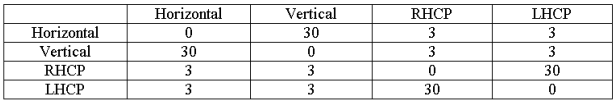The following pictures shows the Vertical, Horizontal and Circular polarization.

 Vertical polarization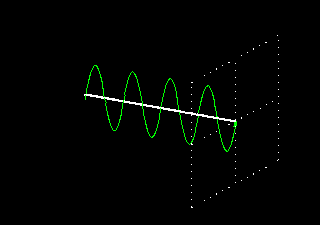Horizontal polarization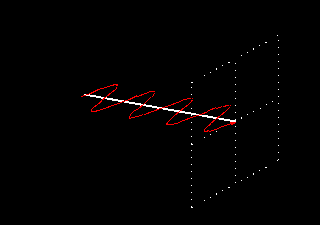Left Hand Circular Polarization(LHCP)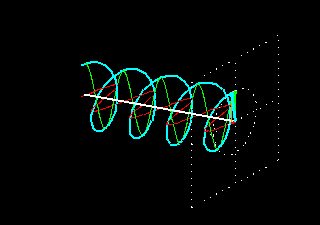Right Hand Circular Polarization(RHCP)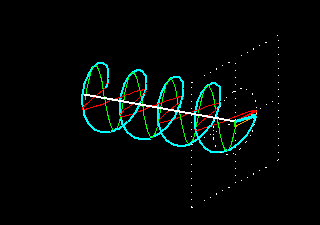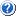A common question among Radio-Amateur operators is : how is possible to achieve Circular polarization?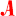In practice, Amateur Satellite operators are using two basic methods:

Helical antennas and crossed-Yagis antennas.

Helical antennas easily offer the wanted circular polarization, depending on how they are constructed. In practice it's a large air-coil onto a boom, where ClockWise (CW) winding gives RHCP and Counter ClockWise gives LHCP. Sometimes however the polarization of the Helical antenna is a disadvantage as it's impossible to invert the polarity. If the Helical antenna is CW gives RHCP. The CCW Helical gives LHCP.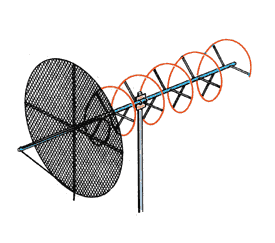Typical Helical antenna:as you can see, this Helical has CW (ClockWise) winding.Thus, this antenna generates RHCP.

This page will describe mainly the cross-Yagi method. Reporting the term "Cross-Yagis", we mean two identical Yagi-antennas where the first one is placed in Vertical position and the second in Horizontal position. In any case the angle between these two antennas must be always 90°, so its also possible to place these antennas on "X" arrangement, the first one with angle of 45° and the second with angle 135° vis-a-vis to "CrossBoom". The picture below shows a typical "X" arrangement with VHF & UHF "cross-Yagi" antennas in order to cover the LEO Satellites.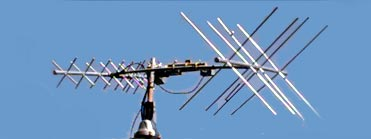The "X" arrangement has a small advantage for a simple reason: the metallic CrossBoom does not affect the antenna's efficiency in contrast with the V-H arrangement (V-H = Vertical-Horizontal), where the CrossBoom is between Horizontal-antenna's elements. That is unwanted ! The figure below shows what happens with the V-H arrangement: half of the horizontal elements are "shadowed" by the CrossBoom.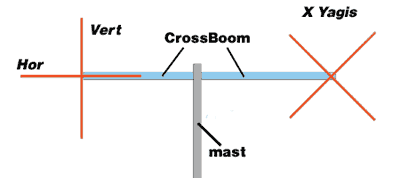But, if the CrossBoom is made from non metallic material (like Wood, PVC etc), there is no significant effect between the CrossBoom and the Horizontal-antenna, so in that case it is possible to mount the two antennas with Vertical and Horizontal classical arrangement.
On the other hand, the V-H arrangement offers a small advantage in case you need to have a "switchable" antenna system in order to change polarization between Vertical (for mobiles), Horizontal (DXing) and Circular polarization (Satellites). The V-H arrangement needs a minimal number of coaxial Relays, in order to be able to make this system "switchable" between Vertical-Horizontal-RHCP polarization, in contrast with the "X" arrangement, which needs much more Coaxial-relays (and phase-stubs).OK, now how to connect these two crossed Yagi antennas? in parallel?Well, Yes and ... No ! To generate Circular polarization with "Crossed-Yagis" two basic requirements are needed:

a) we need to "match" these 2 antennas
b) we need a "phase delay" in order to generate Circular polarity (details below).

Analyzing "a", it's obvious that If we have two antennas which will be connected between each-other, we need a better way than simply connect them in parallel.
The reason for this is simple: if two antennas of 50 Ohms will be connected in parallel, the Impedance at the connection point is 50/2=25 Ohms. That is a problem, as you will have a mismatch with the Coaxial cable, Transmitter etc. Under this condition the SWR will be 2:1.
Still the goal is to connect the two antennas in parallel in such a way that the joint impedance matches with the coax cable from the RX/TX in the shack. As the coax cable is 50 Ohms we need 50 Ohms as well at the connection point of the two antennas.
That means each antenna should be 100 Ohms because then the impedance at the connection point will be 100/2 = 50 Ohms.

For this reason we use "Matching Stubs" between the two antennas and the common Coaxial cable which going down to the Shack .What is a "matching Stub" ?A "Matching Stub" is a piece of Coaxial cable with appropriated calculation in impedance (Z), overall length etc., which acts as a kind of "Impedance transformer" between 2 known but unequal impedances (Z1, Z2). With the "Quarter Wave" formula, it is possible to calculate the intermediate "Z" of a "Quarter Wave" () coaxial piece, in order to be able to match 2 different impedances.

Quarter Wave formula =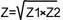A mere example: I have an antenna with Z=50 and I need as a termination-point an impedance of 100 Ohm. Is this possible?

Yes, by using a "Quarter-Wave" coaxial cable with different (intermediate) Z, in order to "match" the Antenna's Z (50 Ohm) to a wanted "termination" Z (100 Ohms).

In this case we have:

 Z = Unknown Z Z1 = Antenna Z Z2 = Termination Z

Z1 & Z2 are known. Antenna's Z = 50, Termination Z = 100 Ohms.... so the formula will be:

Z=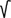Z1 * Z2 => Z=50 * 100 => Z=5000 => Z= 70.7 Ohms

Voila ! the new impedance is 70.7 OhmsOK, we found a new Z=70.7 Ohms... but what to do now with that new "Z" ?As we said before, by using a "Quarter Wave" piece () of coaxial cable with this Z-value (70.7 Ohms), it is possible to match the 50 Ohms antenna to a termination of 100 ohms. But it's very important to keep in mind that, we are talking here for "Electrical Quarter Wave".

"Electrical Quarter Wave" (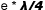) means, a Quarter Wave coaxial piece reduced in length by the Velocity Factor (VF) of Coaxial cable due to reduced propagation-speed of electrical current into Coaxial cable vis-a-vis to the propagation-speed in "free space". The VF of every Coaxial cable is known from the related tables of the manufactures. For example, the VF of RG213, RG11 is 0.66.OK, now how to calculate the whole necessary Coaxial's piece in order to match the 50 Ohms with the 100 Ohms?The method in order to solve this problem is:
1) we choose the type of Coaxial. A coaxial cable with an impedance of 70.7 Ohms does not exist. The closest impedance of Coaxial cables is 75 Ohms, like RG11, RG59 etc. In practice the "mismatch" between 70.7 and 75 Ohms is very small, so the RG11 is perfect for this job. Suppose we choose RG11, the VF = 0.66.

2) We need to know the Wavelength () of the operation frequency. The formula is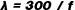, where f is the operation frequency in MHZ. For example, in 2m Band the middle-point of Satellite subzone (145.8 - 146.0 MHz) is 145.9 MHZ, so the formulais:= 300 / 145.9 = 2.056 m

3) Now we divide theby 4, in order to have the:= 2.056 / 4 =>= 0.514 m (or 51.4 cm)

4) Now we know the length of "Quarter Wave" () for 145.9 MHz.
So the "Electrical Quarter Wave" () is:* VF

The VF for RG-11 is also known (0.66), so the= 0.514 * 0.66 =>= 0.339 m

CONCLUSION: between 2 different impedances of 50 & 100 Ohms, a piece of "Electrical Quarter Wave" () of 75 Ohms (designated as "Matching-Stub") is enough, to "match" these two unequal impedances (see following picture).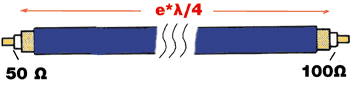OK fine, we learned how to match a 50 Ohms impedance to a 100 Ohms impedance. But how is it possible to connect two 50 Ohms Yagis with 50 Ohms Coaxial? still wondering..The connection of two Yagi-antennas following the abovementioned requires a slightly different approach:

Each antenna is connected to a common-point by using a piece of "Electrical Quarter Wave" () Coaxial cable of 75 Ohms (Matching Stub), in order to transform each 50 Ohms antenna up to 100 Ohms and the two are placed in parallel to produce 50 Ohm again. The following picture shows this combination. On the left picture, the "up" & "down" points on the edges of each 75 Ohms Coaxial piece are connected to 50 Ohms antennas. At the opposite edge of each Coaxial we have 100 Ohms impedance. If these 100 Ohms impedance-points are connected in parallel, the new impedance will be 50 Ohms (100 / 2 = 50), so at this point the joint impedance matches with the usual 50 Ohms Coaxial cables (like RG213, H100, AirCom etc) up to the Shack. That's all !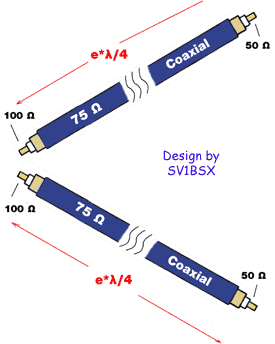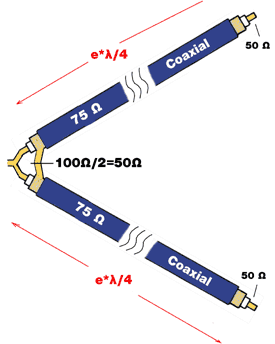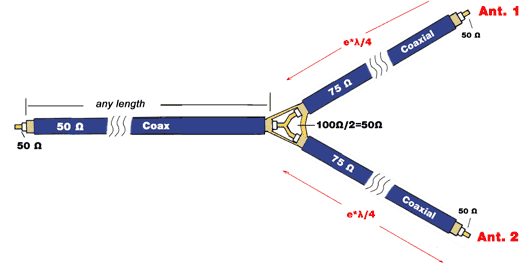Keep in mind, the same approach is used also for the "stacking" of Yagi-antennas, Collinear dipoles etc. The "Matching Stub" is a common method in order to match 2 (or more) antenna's identical of impedance . So, at this point there is nothing unusual with "Crossed-Yagis". They are just two identical Yagi antennas connected to each other in order to have the optimum "matching". The only difference with Crossed Yagis is that, they are NOT both in Horizontal (or Vertical) polarization like stacked-Yagis, but the first one is in Horizontal and the second in Vertical polarization.

Closing the "Matching Stubs" chapter, the picture below shows a few details about preparing of coaxial piece, in order to be able to function as correct "Matching Stub" ().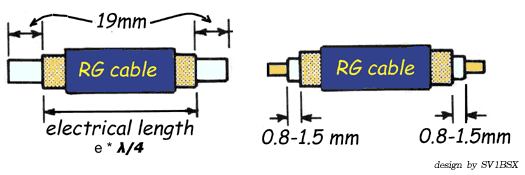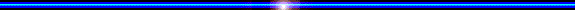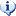...about Phase delay

The second requirement in order to generate Circular Polarization (except "Matching" ) from two Crossed-Antennas is a very interesting and sometimes difficult case. We are talking about the "Phase Delay".
As mentioned in the previous chapter, the Satellite Amateur Radio communication Service basically uses RHCP (Right Hand Circular Polarization) and sometimes LHCP (Left Hand Circular Polarization). But, even the world's top telecommunications engineers got this one wrong on the first transatlantic TV broadcast via Telstar commercial satellite !

First, in order to understand the relationship between Linear, Circular and Elliptical polarization correctly, it is necessary a few words about the theory... so:

 Theoretically, linear and circular polarization are special cases of elliptical polarization. Consider 2 electrical vectors at right angles to each other. The frequencies are the same, but the magnitudes and phase angles can vary. If either one or the other of magnitudes is zero, under this condition the polarization is LINEAR. If the magnitudes are the same and the phase angle between the 2 vectors (in timebase) is exactly 90 degrees, under this condition we have CIRCULAR polarization. Any other combination between these two limits gives ELLIPTICAL polarization.

From this theory, if we talking about Circular polarity is obvious that, its easier to achieve.... Elliptical polarization, than the wanted Circular ! Is high enough just... a small fault (incorrect "phase stamp", bad "matching" etc) as the Circular polarization happens only when the two electrical vectors have 90° difference in phase, all the rest cases give Elliptical and Linear polarization!
Thus it's clear enough , in order to be able to generate in a crossed-Yagis antenna system Circular poalarization, we need to insert into the first antenna a "Phase Delay" precisely equal with 90° vis-a-vis to the second antenna (or vice-versa).How is it possible to insert this necessary "90° phase delay" into crossed-Yagis?In practice this can be done by using two methods:

 1) Electrical method 2) Physical method

Analyzing the "Electrical method", I will not extend in complicated and sometimes difficult theories about "Phase", delay-lines etc. Just keep in mind the fundamental rule of "transmission lines":

a piece ofCoaxial cable produces a "Delay" in Phase equal to 90°
(recognised here as "Phasing Stub").

The following picture shows clearly enough what happens in the above mentioned circuit which has been used in order to connect two identical Yagi-antennas.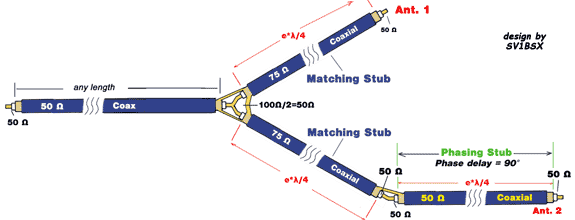As you can see in the picture above, in practice this system includes the arrangement that has been described in the previous chapter about "Matching Stubs". In addition, the necessary "Phasing Stub" in series with ANT-2 has been added. Thus, the feeding of this antenna is "delayed" in phase by 90°, in order to be able to generate the wanted Circular polarization. That's all !OK but what impedance should this "Phasing Stub" be? 75 or 50 Ohms?Definitely 50 Ohms ! This piece of Coaxial is working as a "90° Phase Delay - Stub", not as a "Matcing-Stub". So it must be equal in Ohms with the Antenna's impedance. Don't forget that. Also the overall length of it is also "Electrical", that means* VF, so we talking also about "Electrical Quarter Wave" ().

The calculation of this "Stub" is similar to the "Matching Stub" as above mentioned, so for the Satellite-Subzone (145.9 MHz) a " 90° Phase-Stub" in practice has an overall length = 0.339 m. (or 33.9 cm). The basic difference from "Matching Stub" is that the Coaxial cable that used as "Phase-Stub" is 50 Ohms.
So, keep in mind that: we use 50 Ohmsfor "90° Phasing Stubs", 75 Ohmsfor "Matching Stubs".OK... is it possible to control the RHCP - LHCP polarization in crossed-Yagis?Yes.. depending on the feeding of the two dipoles of cross-Yagi antennas. The following picture shows clearly enough how it is possible to generate RHCP or LHCP ( rear view of dipoles).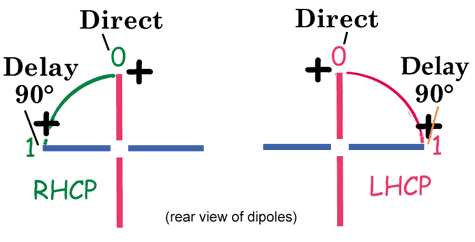The "+" shows the connection of the centre-conductor of each Coaxial cable. The "0" point indicates the direct feeding of the Vertical Dipole (ANT.1) and the "1" indicates the "delayed" feeding of the Horizontal Dipole (ie through a "phase-stub" in ANT.2).
Its obvious that, in practice is high enough just the simple changing of the Coaxial's centre-conductor feeding between left or right part of Horizontal dipole, in order to change the polarization between RHCP & LHCP.Well, now I am really confused ! The left picture above shows the generation of RHCP, but due to "0" starting-point and continue to the the delayed-point "1", this circularity (rotation) is definitely CCW ! Why RHCP polarization? Are you sure?Good observation ! Well, you are right but the antenna beams to the opposite side, the "Front side" (the side where the director-elements are placed). So looking to the left-picture's Dipole from rear view, the circularity seems to be CCW. But if you turn your self to the front-side of antenna, the circularity is just the opposite (CW) !
Always the RHCP (or LHCP) polarization is recognisable by looking the "antenna" from the front side, the beaming-side. In other words the "antenna" and the "observer" are always "face to face". So the pictures above are both 100% correct.Seems to be quite easy ! Is it also the most simple method ?Theoretically yes, but in practice the "Electrical method" mentioned above can cause a lot of problems !
For example, the feeding-point (dipole) into a Yagi antenna is not a "pure" carbon 50 Ohms resistor, but has an impedance. For example, according to the capacitive or inductive behaviour (-j or +j) of Yagi-antennas, the added "Phasing-Stub" sometimes significantly changes the equal amount of "Power-feeding" between the two crossed-antennas. Also, an incorrect "Matching-Stub" or "Phase Stub" is another bad scenario. In practice many Satellite-operators don't yet have the experience and the "Know-How" in order to be able to make "Stubs" with the necessary accuracy.
So, conclusively if two crossed Yagi-antennas have un-equal feeding, especially due to an additional piece of "Phase Stub" which transforms the impedance from one of the antennas away from 50 ohm, at the point where the two Coaxial cables are joined from the two individual antennas the impedance will be unequal. Therefore the Power is not split equally between the two cross-antennas and the polarization becomes Elliptical !
The worst scenario is that, several Sat-operators just they don't realize the abovementioned ! They suppose that they have Circular polarization, but have they? That is a good question !

For this reason it is much easier, especially for inexperienced Sat-operators (rookies), to make a cross-Yagi antenna system for Circular polarization, by using the "Physical Method".

Physical method

This method differs in the "import-technique" of Phase's Difference. In the previous chapter was described how in practice it's possible to feed the 2nd antenna with 90° "Difference in phase" vis-a-vis to 1st antenna, by using acoaxial piece (50 Ohms) that works as "Phase Stub".

The "Physical Method" following a different technique in order to avoid the use of coaxial piece for the necessary 90° phase's difference. Here the "Phase Difference" has been achieved by "shifting" (moving) each element of the 2nd (Horizontal) antenna exactly one Quarter-Wavelength () forward in space, vis-a-vis to each element of 1st (Vertical) antenna onto the common Boom.
The following picture shows this arrangement clearly enough. As you can see, the space between VD1(Vertical dipole) and HD1 (Horizontal dipole) is.
Just the same happens between VE1 (Vertical Element-1) & HE1(Horizontal Element-1), VE2 & HE2 etc.Thus, with this method it is not necessary to use an additional piece of Coaxial-cable as a "Phasing Stub". The construction is simple, it is enough just a "Matcing-Stub" system in order to connect (and split) the feeding Power between the two antennas. However the great advantage is not the more simple construction but:

"Power splitting" between the two antennas is now equal, as the two "Matching Stubs" (2 Coaxial pieces 75 Ohms) in the splitting have precisely equal length and therefore give identical transformation of antenna's impedance. So, the basic disadvantage of crossed Yagis with a Coaxial's "Phasing Stub" now has been completely eliminated !the "connection-points" on Coaxial cables between "Matching" & "Phasing Stubs" are reduced (in practice the "Phase Stub" does not exist any longer), that means much lower number of connectors (or soldering points), especially important on UHF & higher frequencies where the "Loss" increases by using additional connectors, even in case where the connectors are N-type.The "splitting" between the two antennas is easier, it can be achieved now just by using a commercial Splitter/Combiner unit (1 to 2 antennas) that is easily available on the market. Thus, it is possible to avoid the calculation and construction of "Matching & Phasing Stubs" with Coaxial-pieces.The"space" between the antennas is a physical, pure number (=300/F and then divide /4, where F=operating frequency). Thus is not necessary to involve the "Velocity factors" of Coaxial-cables and other tenebrous (for rookies) "Square roots", complex equations & norms into the calculations. For example, by using the above mentioned formula, for VHF satellite subzones the "shifting" space () is:=300/F =>=300/145.9=>= 2.056m=2.056/4=>= 0.514m or 51.4cm

For UHF respectively theis 17.2 cm (for 435-436 MHz).

Finally, the following pictures show Right-Hand and Left-Hand Circular polarization depending on the connection of the centre-conductor of the Coaxial cable onto the Horizontal dipole.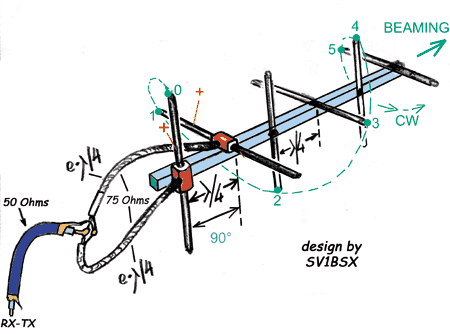On the picture above, the centre conductor of the Coaxial-cable is connected to the left part of the Horizontal dipole (as shown the "+"). Thus, the polarity is RHCP (CW).

The picture below shows the opposite Circular polarization (LHCP or CCW). Here the centre-conductor of the Coaxial-cable is connected to the right-part of the Horizontal dipole, in order to generate LHCP (CCW) Circular polarization.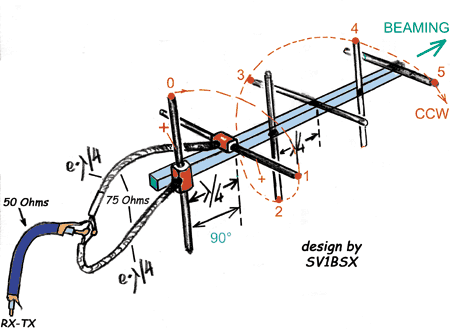In both cases the centre conductor of the Vertical-dipole's Coaxial-feeder is connected to the "upper" section of the dipole as shown by "+".

 Have fun with Satellites!Mak SV1BSX (April 2006)

Related pages:
 SV1BSX © CopyrightAll rights reserved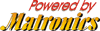No part of this page may be reproduced, dublicated, printed & copied without permission.For HAM-Radio PERSONAL USE ONLY